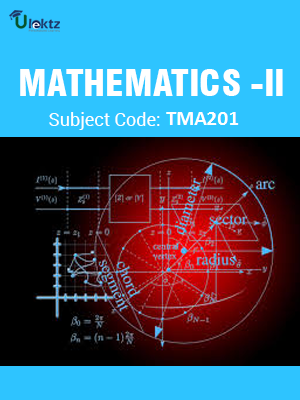uLektz apps

•My WalletMy Order
•My Profile
•My Connections
•My Books
•My Videos
•My Tests
•My Calender
•My Messages
•My Shopping Cart
•My Orders
•Account Settings
•Help

uLektz apps

# Book Details# MATHEMATICS-II

Course Code:TMA201

Author:uLektz

University:

Regulation:2010

Categories:Engineering Mathematics

Format :ePUB3 (DRM Protected)

Type :eBook

FREE

Description :MATHEMATICS-II of TMA201 covers the latest syllabus prescribed by Uttarakhand Technical University for regulation 2010. Author: uLektz, Published by uLektz Learning Solutions Private Limited.

Note : No printed book. Only ebook. Access eBook using uLektz apps for Android, iOS and Windows Desktop PC.

##### Topics
###### UNIT - I DIFFERENTIAL EQUATIONS

1.1 Ordinary differential equations of first order

1.2 Exact differential equations

1.3 Linear differential equations of first order

1.4 Linear differential equations of nth order with constant coefficients

1.5 Complementary functions and particular integrals

1.6 Simultaneous linear differential equations

1.7 Solutions of second order differential equations by changing the dependent and independent variables

1.8 Method of variation of parameters

###### UNIT - II LAPLACE TRANSFORM

2.1 Laplace transform

2.2 Existence theorem

2.3 Laplace transform of derivatives and integrals

2.4 Inverse Laplace transform

2.5 Laplace transform of periodic function, Unit step function

2.6 Convolution theorem

2.7 Applications to solve simple linear and simultaneous linear differential equations

###### UNIT - III INFINITE SERIES

3.1 Introduction, Sequences

3.2 Series: Convergence

3.3 Series of positive terms

3.4 Comparison tests

3.5 Integral tests

3.6 Comparison of ratio‘s

3.7 D‘Alembert ratio test

3.8 Raabe‘s test

3.9 Cauchy root test

3.10 Alternating series: Lebnitz rule

3.11 Power series

3.12 Uniform convergence

3.13 Weierstrass‘s M-test

3.14 Properties of uniformly convergent series

###### UNIT - IV FOURIER SERIES AND PARTIAL DIFFERENTIAL EQUATIONS

4.1 Periodic functions

4.2 Trigonometric series

4.3 Fourier series of periodic function

4.4 Euler‘s formula

4.5 Functions having arbitrary period

4.6 Change of intervals

4.7 Even and odd functions

4.8 Half range sine and cosine series

4.9 Introduction to partial differential equations

4.10 Linear partial differential equations with constant coefficients of second order and their classifications: parabolic, hyperbolic and elliptic with illustrative examples

###### UNIT - V APPLICATIONS OF PARTIAL DIFFERENTIAL EQUATIONS

5.1 Method of separation of variables for solving partial differential equations

5.2 One dimensional wave equation

5.3 Laplace equation in two dimensions

5.4 Heat conduction equations of one dimension and two dimension

### Related Books